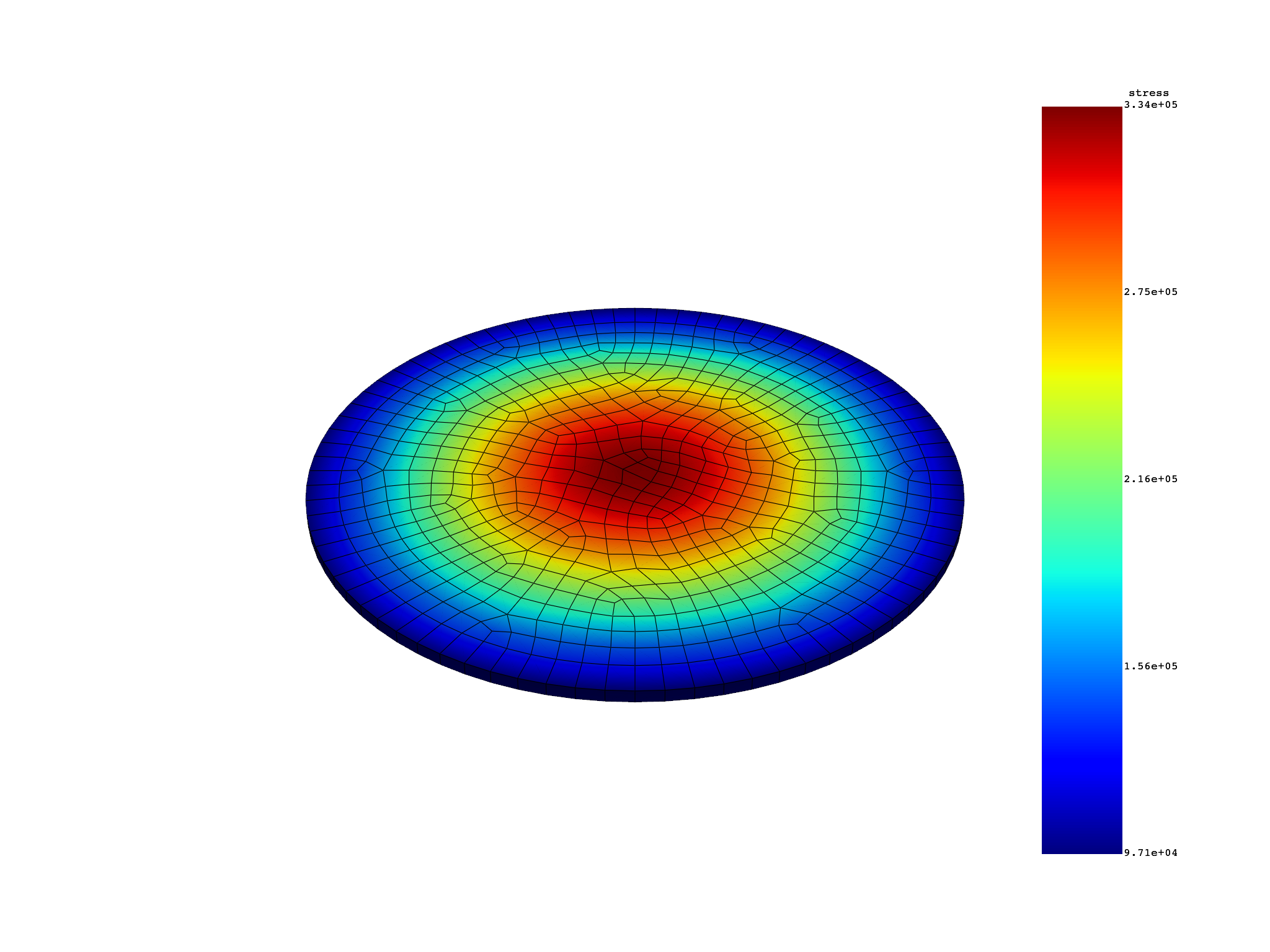# Harmonic analysis#

This example shows how you can post-process a result file for a harmonic analysis using PyDPF-Post.

## Perform required imports#

Perform required imports.

```from ansys.dpf import post
from ansys.dpf.post import examples
```

## Get `Solution` object#

Get the `Solution` object. This example loads a result file for an harmonic analysis computed in Ansys Mechanical.

```example_path = examples.download_all_kinds_of_complexity()

print(solution)
```
```Harmonic Analysis Solution object.

Data Sources
------------------------------
DPF  DataSources:
Result files:
result key: rst and path: /opt/hostedtoolcache/Python/3.8.16/x64/lib/python3.8/site-packages/ansys/dpf/core/examples/complex.rst
Secondary files:

DPF Model
------------------------------
Harmonic analysis
Unit system: MKS: m, kg, N, s, V, A, degC
Physics Type: Mechanical
Available results:
-  displacement: Nodal Displacement
-  reaction_force: Nodal Force
-  stress: ElementalNodal Stress
-  elemental_volume: Elemental Volume
-  stiffness_matrix_energy: Elemental Energy-stiffness matrix
-  artificial_hourglass_energy: Elemental Hourglass Energy
-  thermal_dissipation_energy: Elemental thermal dissipation energy
-  kinetic_energy: Elemental Kinetic Energy
-  co_energy: Elemental co-energy
-  incremental_energy: Elemental incremental energy
-  elastic_strain: ElementalNodal Strain
-  elemental_heat_generation: Elemental Elemental Heat Generation
-  structural_temperature: ElementalNodal Temperature
-  electric_potential: Nodal Electric Potential
-  electric_flux_density: ElementalNodal Electric flux density
-  electric_field: ElementalNodal Electric field
------------------------------
DPF  Meshed Region:
4802 nodes
657 elements
Unit: m
With solid (3D) elements
------------------------------
DPF  Time/Freq Support:
Number of sets: 1
With complex values

Cumulative     Frequency (Hz) LoadStep       Substep        RPM
1              343478.200000  1              1              0.000000

This may contain complex results.
```

## Get `Result` objects#

### Get displacement result#

Get the displacement `Result` object. It contains a field for real values and a field for imaginary values.

```disp_result = solution.displacement()
disp = disp_result.vector
```

### Check number of fields#

Check the number of fields.

```disp.num_fields
```
```2
```

### Get data from field#

Get data from a field.

```disp.get_data_at_field(0)
```
```DPFArray([[ 2.65783929e-09, -5.98949034e-10,  8.34267891e-11],
[ 2.63846617e-09, -3.00204960e-10,  8.27306877e-11],
[ 2.50179982e-09, -2.86371281e-10,  6.29386453e-11],
...,
[-1.70840238e-09, -2.73504676e-09,  3.48706947e-11],
[-1.57038405e-09, -2.71125223e-09,  6.79105278e-11],
[-1.57311157e-09, -2.71904943e-09,  0.00000000e+00]])
```

### Get maximum data value over all fields#

Get the maximum data value over all fields.

```disp.max_data
```
```DPFArray([[2.76941713e-09, 2.76940199e-09, 4.10914311e-10],
[6.53706736e-13, 6.53416337e-13, 9.25220948e-14]])
```

### Get minimum data value over all fields#

Get the minimum data value over all fields.

```disp.min_data
```
```DPFArray([[-2.76946046e-09, -2.76952549e-09,  0.00000000e+00],
[-6.53727285e-13, -6.53452004e-13, -1.66091913e-13]])
```

### Get maximum data value over targeted field#

Get the maximum data value over a targeted field.

```disp.get_max_data_at_field(0)
```
```DPFArray([2.76941713e-09, 2.76940199e-09, 4.10914311e-10])
```

### Get minimum data value over all fields#

Get the minimum data value over all fields.

```disp.get_min_data_at_field(0)
```
```DPFArray([-2.76946046e-09, -2.76952549e-09,  0.00000000e+00])
```

## Get stress result#

Get a stress result that deals with amplitude. It contains a field for real values and a field for imaginary values.

```stress_result = solution.stress()
```

### Check if support has complex frequencies#

Check if the support has complex frequencies.

```stress_result.has_complex_frequencies()
```
```True
```

### Get tensor result#

Get the `Result` for a tensor.

```stress = stress_result.tensor

# Check number of fields
# ~~~~~~~~~~~~~~~~~~~~~~
# Check the number of shell and solid elements in distinct fields. Shell and
# solid elements are in distinct fields. Thus, you have four fields: the
# solid-real one, the solid-imaginary one, the shell-real one, and the
# shell-imaginary one.

stress.num_fields
```
```2
```

### Get shell field#

Get the shell field.

```shell_field = stress
shell_field.shell_layers
```
```<shell_layers.nonelayer: 5>
```

### Get solid field#

Get the solid field.

```solid_field = stress
```

### Plot amplitude contour#

Plot the amplitude contour.

```amplitude = stress_result.tensor_amplitude
amplitude.plot_contour()
```# Get elastic strain result#

Get an elastic strain result that deals with phase. It contains a field for real values and a field for imaginary values.

```elastic_strain_result = solution.elastic_strain()
elastic_strain = elastic_strain_result.tensor
# shell and solid elements are in distinct fields.
elastic_strain.num_fields
```
```2
```

Define the phase. The phase must be a float value. The unit is degrees.

```es_at_phase = elastic_strain_result.tensor_at_phase(39.0)
es_at_phase.max_data
es_at_phase.num_fields
real_field = elastic_strain_result.tensor_at_phase(0.0)
img_field = elastic_strain_result.tensor_at_phase(90.0)
```

If the result file contains results, you can use this method to get the elastic strain result.

```print(solution.elastic_strain())
```
```Complex tensor object.

Tensor object.

Object properties:
- location   : Nodal

Complex elastic strain object.
```

Total running time of the script: ( 0 minutes 0.626 seconds)

Gallery generated by Sphinx-Gallery